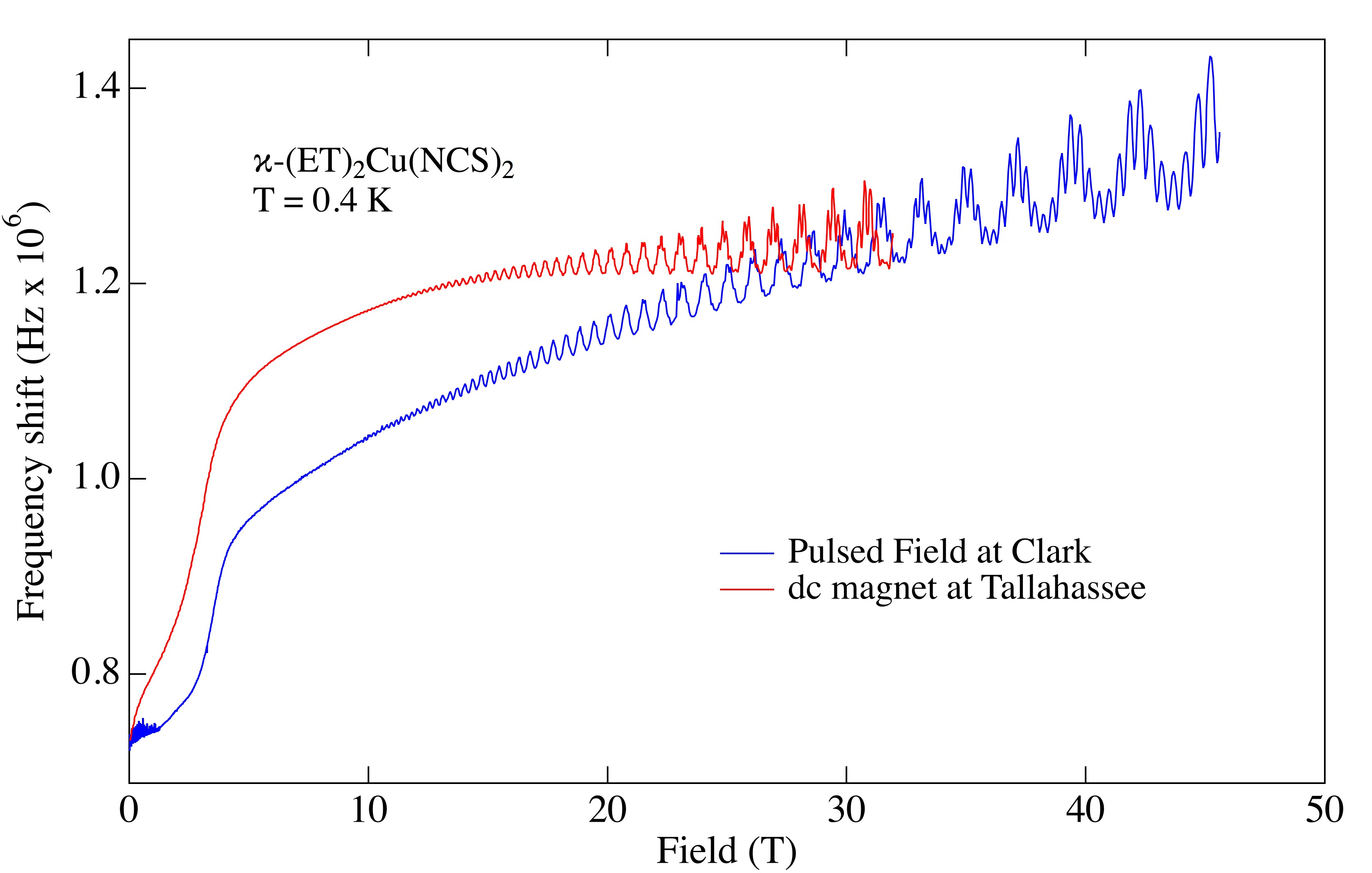## Magnetic Oscillations in Metals.

#### David Howe and Chuck Agosta - Clark University

Magnetic oscillations are variations of a measured parameter as a function of an applied magnetic field, such as resistance, magnetization or specific heat due to the quantization of the energy levels of electron cyclotron orbits. The main feature of the oscillations is their periodicity if plotted versus inverse magnetic field (1/H). Fig 1 shows an example of magnetic oscillations in one of the organic materials we have studied, κ-(BEDT-TTF)$_2$Cu(NCS)$_2$ Fig.2 (coming soon!) shows the same resistance data plotted versus inverse magnetic field.A comparison of quantum oscillations in resistance measured via rf penetration depth subject to pulsed and dc magnetic fields. This material has a fundamental orbit from a nearly cylindrical Fremi surface and a higher frequency due to tunneling of electrons to an additional part of the Fermi surface. The frequency of an observed oscillation is connected to a particular extremal area of the Fermi surface through the following formula:
\begin{equation} A_m = \frac{2 \pi e}{\hbar}F,\label{eq:Am} \end{equation} where $A_m$ - extremal area of the Fermi surface perpendicular to applied magnetic field, $e$ - electron charge, $F$ - frequency of oscillations. Units in (1) are in the SI system. All right, you may want to say, but that does not explain, why would we observe the oscillations in first place? The very simplified explanation follows.
First of all, the motion of electrons is quantized in a magnetic field. In other words, when a magnetic field is applied, electrons start to move in circles, but not all the possible radii are permitted. The allowed energies are described by: \begin{equation} E_n = \frac{p^2_z}{2m}+\frac{\hbar eH}{m}\Big(n+\frac{1}{2}\Big),\label{eq:En} \end{equation} where $E_n$ is the energy of an electron, $H$ is the magnetic field, $m$ is the mass of an electron, $n$ is an integer number, $p_z$ is the projection of electron's momentum in the direction of magnetic field. Because this fundamental formula ties energy and momentum from here on we will operate in momentum space instead of our normal space of 3 coordinates.
As follows from (2), for each given $n$ the energy is proportional to a magnetic field, in other words, diameters of orbits in momentum space will grow as the magnetic field is increased.

That is nice to know, you would say, but there is still nothing to oscillate here... That's right, to make things periodically change we will have to dig a little deeper into Physics of Solid State.

In metals there is a particularly important energy value, the Fermi Energy, which is the highest occupied electron energy level. Similar to the chemical properties of atoms, it is the highest occupied energy levels that determine most of the properties of a system. In this case, it is the density of states, the number of available quantum states per energy interval that detrmines most properties of a metal, such as conductivity, magnetic susceptibility or specific heat. As the Landau levels periodically pass through the Fermi Energy the density of states oscillates, it is higher when a Landau level is near the Fermi Energy lower when a Landau level is not near the Fermi energy, and most measurable electronic properties of the material will oscillate due to this effect.
2222
last updated 8 Mar 2019
Webmaster
cagosta@clarku.edu